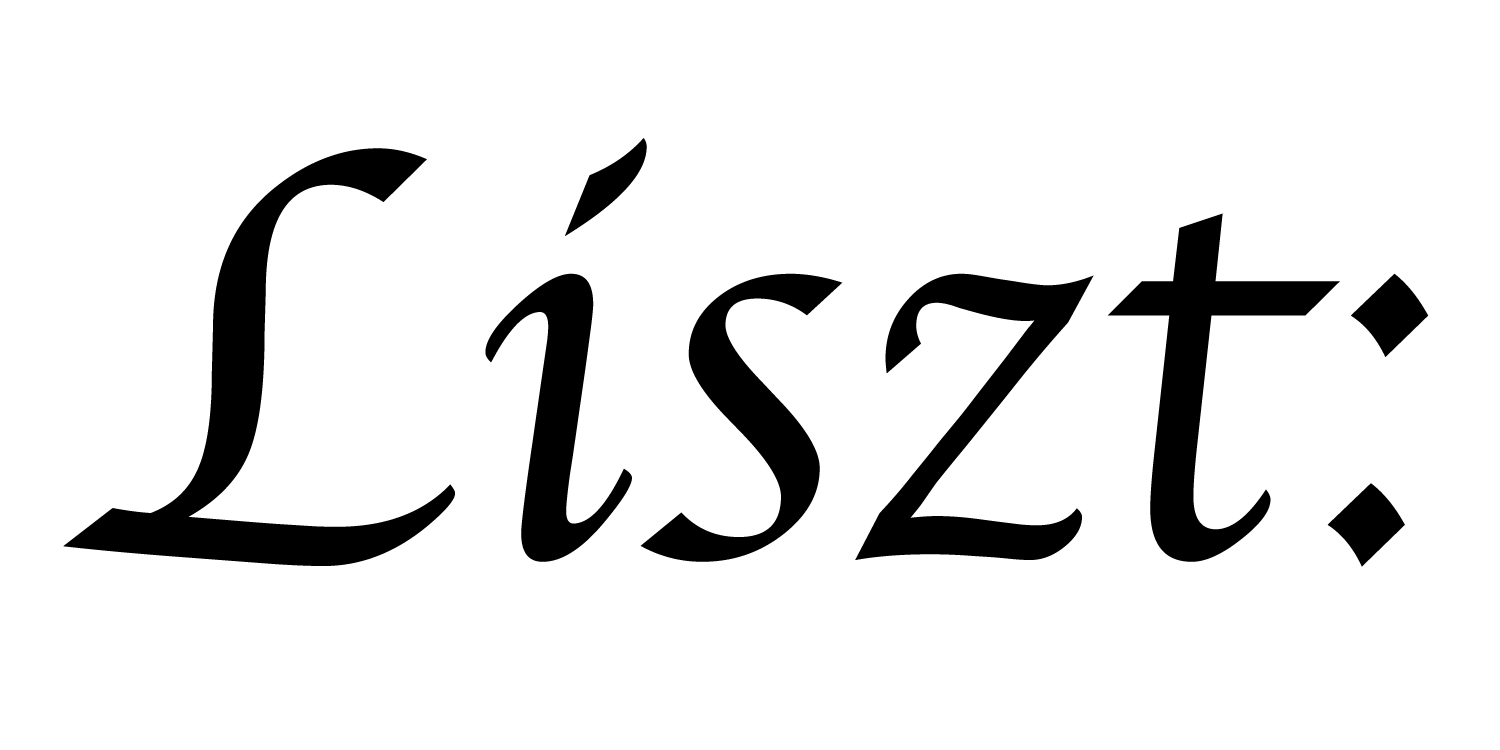# 09: Particle-Grid Coupling

In this tutorial, we’ll look at how to couple two geometric domains together, which is often critical for simulating interacting physical phenomena.

``````import 'ebb'
local L = require 'ebblib'

local GridLib   = require 'ebb.domains.grid'

local vdb       = require 'ebb.lib.vdb'

local N = 40

local grid = GridLib.NewGrid2d {
size   = {N, N},
origin = {0, 0},
width  = {N, N},
periodic_boundary = {true,true},
}
``````

We start the grid-based heat diffusion the same way. For simplicity, we’re now using only periodic boundaries and placing the origin at 0,0

``````local timestep    = L.Constant(L.double, 0.45)
local conduction  = L.Constant(L.double, 1.0)

local function init_temperature(x_idx, y_idx)
if x_idx == 4 and y_idx == 6 then return 1000
else return 0 end
end

local ebb update_temperature ( c : grid.cells )
var avg   = (1.0/4.0) * ( c(1,0).t + c(-1,0).t + c(0,1).t + c(0,-1).t )
var diff  = avg - c.t
c.new_t   = c.t + timestep * conduction * diff
end
``````

The code for doing the heat diffusion itself is more or less unchanged, though we’ve omitted a lot of it for simplicity.

``````local particles = L.NewRelation {
name = "particles",
size = N*N,
}
``````

To model the particles, we don’t need a special domain library. Simply creating a relation to model the particles will suffice. Here we create one particle for each cell in the grid.

``````local particle_positions = {}
for yi=0,N-1 do
for xi=0,N-1 do
particle_positions[ xi*N + yi + 1 ] = { xi + 0.5,
yi + 0.5 }
end
end
``````

We initialize these particles to sit at the center of each cell. (note that we again have to compensate for the difference between Lua’s 1-based indexing and the 0-based indexing in Ebb.)

``````particles:NewField('cell', grid.cells)
``````

In order to connect the two geometric domains together, we create a field of keys referencing the cells from the particles. This connection allows us to encode the concept that a particle is located inside a given cell.

``````grid.locate_in_cells(particles, 'pos', 'cell')
``````

The standard grid gives us a special function that we can use to populate the dynamic `cell` connection. `grid.locate_in_cells()` takes 3 argumetns: 1. a relation with a field of keys to `grid.cells`, 2. the name of a field of that relation holding spatial positions of points/particles and 3. the name of the field of keys pointing into `grid.cells`. Here, we’re calling this function early in order to initialize the values of the `particles.cell` field.

``````local ebb wrap( x : L.double )
return L.fmod(x + 100*N, N)
end

local ebb advect_particle_position ( p : particles )
-- estimate heat gradient using a finite difference
var c   = p.cell
var dt  = { c(1,0).t - c(-1,0).t, c(0,1).t - c(0,-1).t }
-- and move the particle downwards along the gradient
var pos = p.pos - 0.1 * timestep * dt
-- wrap around the position...
p.pos = { wrap(pos), wrap(pos) }
end
``````

We’re going to define the particles’ motion using the gradient of the grid’s temperature field. At each timestep, the particles should move a little bit towards the cooler regions. We do this by first looking up the cell the field is located in and then taking a finite difference approximation of the gradient at that grid cell.

One slight complication is that we need to wrap these coordinates around in order to respect the periodic boundary. To keep the code clean, we use another ebb function to define that wraparound.

``````local ebb visualize_particles ( p : particles )
vdb.color({ 1, 1, 0 })
var p2 = p.pos
vdb.point({ p2, p2, 0 })
end
``````

Rather than visualize the underlying grid, we plot the particles.

``````for i=1,360 do
grid.cells:foreach(update_temperature)
grid.cells:Swap('t', 'new_t')

grid.locate_in_cells(particles, 'pos', 'cell')

vdb.vbegin()
vdb.frame()
particles:foreach(visualize_particles)
vdb.vend()

if i % 10 == 0 then print( 'iteration #'..tostring(i) ) end
end
``````

Finally, our simulation loop is mostly unchanged, except we add two calls: to update the particle positions and to update the connection to the cells.

a part of the Liszt project and PSAAP II center at Stanford University